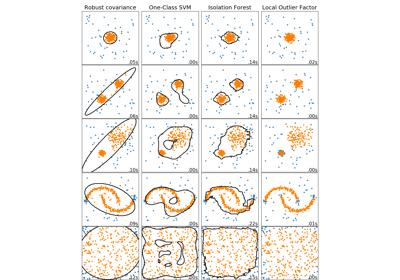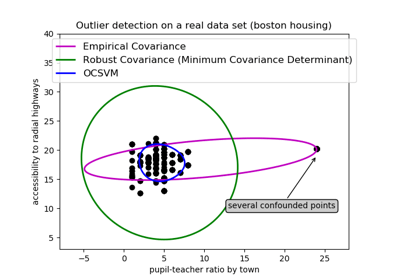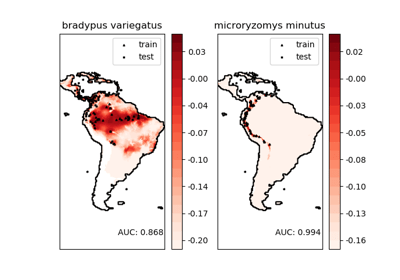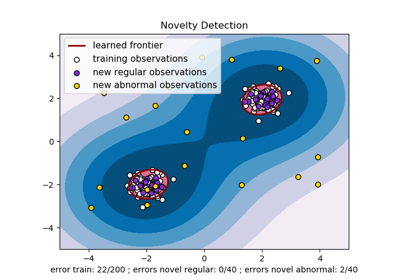/scikit-learn

sklearn.svm.OneClassSVM

class sklearn.svm.OneClassSVM(kernel=’rbf’, degree=3, gamma=’auto_deprecated’, coef0=0.0, tol=0.001, nu=0.5, shrinking=True, cache_size=200, verbose=False, max_iter=-1, random_state=None) [source]

Unsupervised Outlier Detection.

Estimate the support of a high-dimensional distribution.

The implementation is based on libsvm.

Read more in the User Guide.

Parameters: kernel : string, optional (default=’rbf’) Specifies the kernel type to be used in the algorithm. It must be one of ‘linear’, ‘poly’, ‘rbf’, ‘sigmoid’, ‘precomputed’ or a callable. If none is given, ‘rbf’ will be used. If a callable is given it is used to precompute the kernel matrix. degree : int, optional (default=3) Degree of the polynomial kernel function (‘poly’). Ignored by all other kernels. gamma : float, optional (default=’auto’) Kernel coefficient for ‘rbf’, ‘poly’ and ‘sigmoid’. Current default is ‘auto’ which uses 1 / n_features, if gamma='scale' is passed then it uses 1 / (n_features * X.std()) as value of gamma. The current default of gamma, ‘auto’, will change to ‘scale’ in version 0.22. ‘auto_deprecated’, a deprecated version of ‘auto’ is used as a default indicating that no explicit value of gamma was passed. coef0 : float, optional (default=0.0) Independent term in kernel function. It is only significant in ‘poly’ and ‘sigmoid’. tol : float, optional Tolerance for stopping criterion. nu : float, optional An upper bound on the fraction of training errors and a lower bound of the fraction of support vectors. Should be in the interval (0, 1]. By default 0.5 will be taken. shrinking : boolean, optional Whether to use the shrinking heuristic. cache_size : float, optional Specify the size of the kernel cache (in MB). verbose : bool, default: False Enable verbose output. Note that this setting takes advantage of a per-process runtime setting in libsvm that, if enabled, may not work properly in a multithreaded context. max_iter : int, optional (default=-1) Hard limit on iterations within solver, or -1 for no limit. random_state : int, RandomState instance or None, optional (default=None) Ignored. Deprecated since version 0.20: random_state has been deprecated in 0.20 and will be removed in 0.22. support_ : array-like, shape = [n_SV] Indices of support vectors. support_vectors_ : array-like, shape = [nSV, n_features] Support vectors. dual_coef_ : array, shape = [1, n_SV] Coefficients of the support vectors in the decision function. coef_ : array, shape = [1, n_features] Weights assigned to the features (coefficients in the primal problem). This is only available in the case of a linear kernel. coef_ is readonly property derived from dual_coef_ and support_vectors_ intercept_ : array, shape = [1,] Constant in the decision function. offset_ : float Offset used to define the decision function from the raw scores. We have the relation: decision_function = score_samples - offset_. The offset is the opposite of intercept_ and is provided for consistency with other outlier detection algorithms.

Methods

 decision_function(X) Signed distance to the separating hyperplane. fit(X[, y, sample_weight]) Detects the soft boundary of the set of samples X. fit_predict(X[, y]) Performs outlier detection on X. get_params([deep]) Get parameters for this estimator. predict(X) Perform classification on samples in X. score_samples(X) Raw scoring function of the samples. set_params(**params) Set the parameters of this estimator.
__init__(kernel=’rbf’, degree=3, gamma=’auto_deprecated’, coef0=0.0, tol=0.001, nu=0.5, shrinking=True, cache_size=200, verbose=False, max_iter=-1, random_state=None) [source]
decision_function(X) [source]

Signed distance to the separating hyperplane.

Signed distance is positive for an inlier and negative for an outlier.

Parameters: X : array-like, shape (n_samples, n_features) dec : array-like, shape (n_samples,) Returns the decision function of the samples.
fit(X, y=None, sample_weight=None, **params) [source]

Detects the soft boundary of the set of samples X.

Parameters: X : {array-like, sparse matrix}, shape (n_samples, n_features) Set of samples, where n_samples is the number of samples and n_features is the number of features. sample_weight : array-like, shape (n_samples,) Per-sample weights. Rescale C per sample. Higher weights force the classifier to put more emphasis on these points. y : Ignored not used, present for API consistency by convention. self : object

Notes

If X is not a C-ordered contiguous array it is copied.

fit_predict(X, y=None) [source]

Performs outlier detection on X.

Returns -1 for outliers and 1 for inliers.

Parameters: X : ndarray, shape (n_samples, n_features) Input data. y : Ignored not used, present for API consistency by convention. y : ndarray, shape (n_samples,) 1 for inliers, -1 for outliers.
get_params(deep=True) [source]

Get parameters for this estimator.

Parameters: deep : boolean, optional If True, will return the parameters for this estimator and contained subobjects that are estimators. params : mapping of string to any Parameter names mapped to their values.
predict(X) [source]

Perform classification on samples in X.

For an one-class model, +1 or -1 is returned.

Parameters: X : {array-like, sparse matrix}, shape (n_samples, n_features) For kernel=”precomputed”, the expected shape of X is [n_samples_test, n_samples_train] y_pred : array, shape (n_samples,) Class labels for samples in X.
score_samples(X) [source]

Raw scoring function of the samples.

Parameters: X : array-like, shape (n_samples, n_features) score_samples : array-like, shape (n_samples,) Returns the (unshifted) scoring function of the samples.
set_params(**params) [source]

Set the parameters of this estimator.

The method works on simple estimators as well as on nested objects (such as pipelines). The latter have parameters of the form <component>__<parameter> so that it’s possible to update each component of a nested object.

Returns: self

Examples using sklearn.svm.OneClassSVMComparing anomaly detection algorithms for outlier detection on toy datasetsOutlier detection on a real data setSpecies distribution modelingLibsvm GUIOne-class SVM with non-linear kernel (RBF)

© 2007–2018 The scikit-learn developers